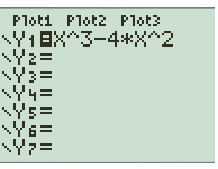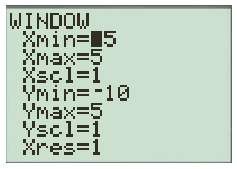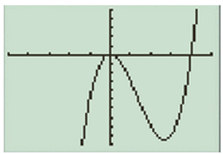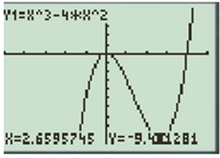# Find the interval of increasing and decreasing of the given function f ( x ) = x 3 − 4 x 2 by using T1-83 calculator.### Precalculus: Mathematics for Calcu...

6th Edition
Stewart + 5 others
Publisher: Cengage Learning
ISBN: 9780840068071### Precalculus: Mathematics for Calcu...

6th Edition
Stewart + 5 others
Publisher: Cengage Learning
ISBN: 9780840068071

#### Solutions

Chapter 2, Problem 53RE
To determine

## Find the interval of increasing and decreasing of the given function f(x)=x3−4x2 by using T1-83 calculator.

Expert Solution

The graph is increasing on (,0],[2.67,) and decreasing on [0,2.67]

### Explanation of Solution

Given information: consider the function f(x)=x34x2

Calculation:

Press the Y= button and enter the function X^3-4*X^2Once this is done on the Window screen

X min =5

X max=5

Y min =-10

Y max=5

This gives a rang that is good for many

Varied graph types

Set the windowThen click the GRAPH button.For finding increase and decrease intervals, click the TRACE button and use the left and right buttons to find values.

So the graph is increase on  to 0, 2.67  to   and decrease from 0.Thus, the graph is increasing on (,0],[2.67,) and decreasing on [0,2.67]

### Have a homework question?

Subscribe to bartleby learn! Ask subject matter experts 30 homework questions each month. Plus, you’ll have access to millions of step-by-step textbook answers!